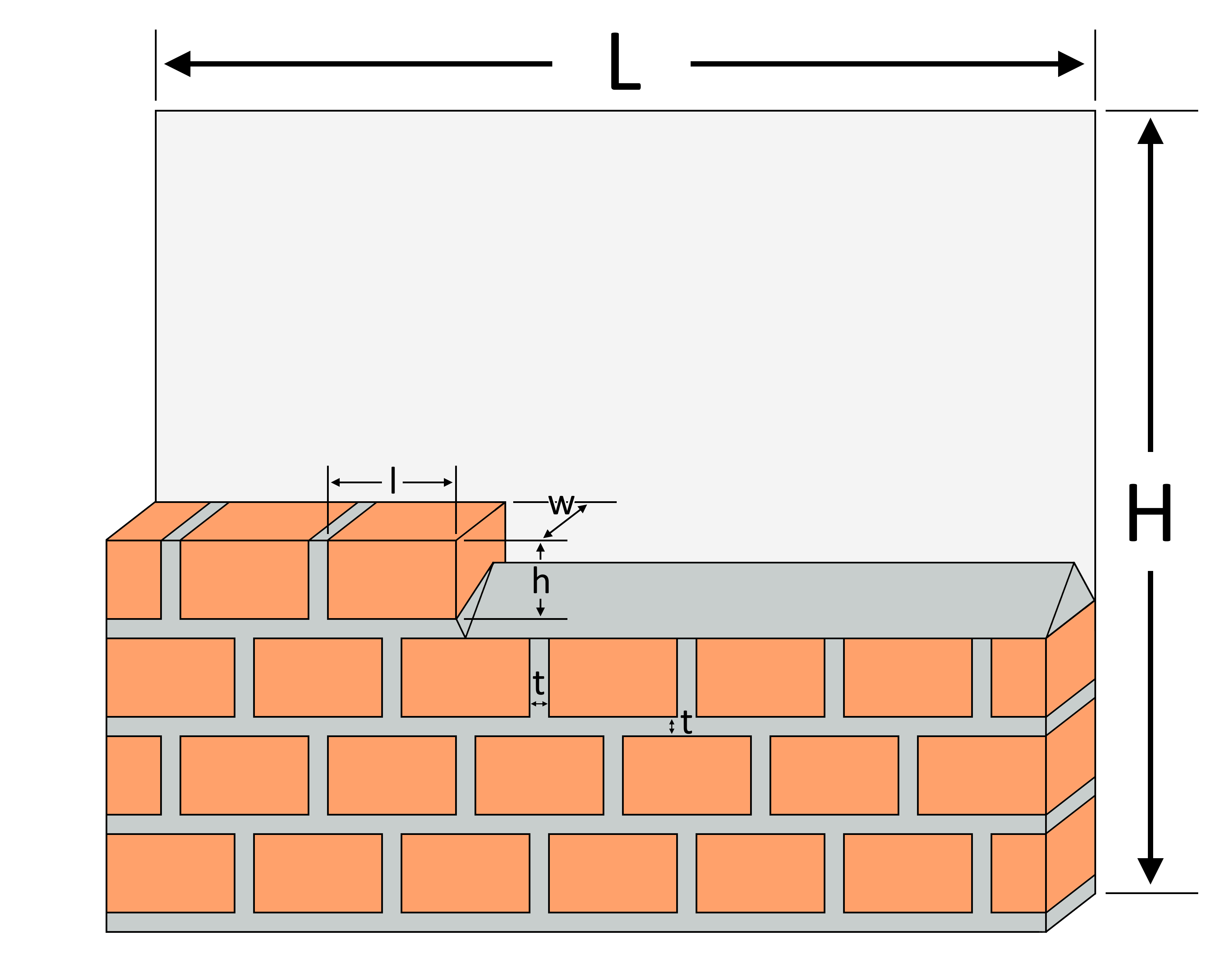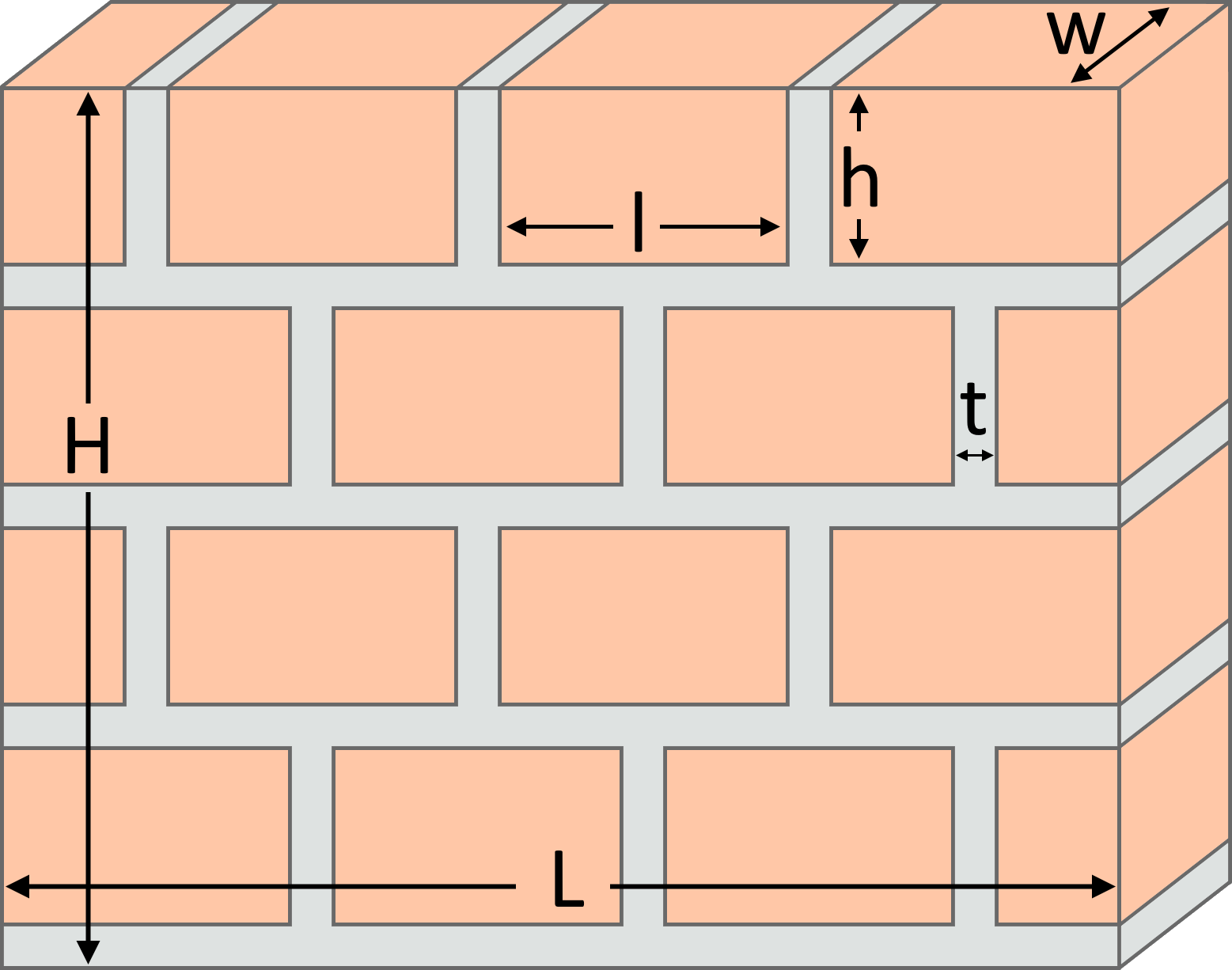# Brick Calculator

Created by Kenneth Alambra
Last updated: Dec 16, 2022

This brick calculator can help you determine how many bricks you need for a brick wall project. With this brick wall calculator, you'll never have to guess how many bricks and how much mortar materials to buy. Keep on reading to learn:

• How to calculate how many bricks you need;
• How much mortar you'll need for your brick wall; and
• How to use this brick calculator.

## How many bricks you need

To find how many bricks you need for any size of brick walls, you can use this very easy-to-follow brick calculation formula that is similar to the formula used in determining the number of tiles:

$\small n = \frac{(L\times H)}{(l + t) \times (h + t)}$

where:

• $n$ is the number of bricks needed;
• $L$ is the length of the wall;
• $H$ is the height of the wall;
• $l$ is the length of the brick;
• $h$ is the height of the brick; and
• $t$ is the thickness of the mortar between the bricks.

Here is an illustration of these dimensions for your reference:🙋 Make sure to measure your brick wall's length along a flat elevation grade for precise calculations.

## How much mortar you need for your brick wall

On the other hand, for brick mortar calculations, you can use this formula:

$\small v = ((L\times H) - (n\times l\times h))\times w$

where:

• $v$ is the volume of mortar needed;
• $L$ is the length of the wall;
• $H$ is the height of the wall;
• $n$ is the number of bricks needed;
• $l$ is the length of the brick;
• $h$ is the height of the brick; and
• $w$ is the width of the bricks.

According to this equation, we need to subtract the total brick area from the total wall area and multiply the difference by the bricks' width to find the required mortar volume. If you know mortar density, you can then estimate its mass using the simple .

## How to use our brick calculator

To use our brick calculator, you have to enter the dimensions of your brick wall, as illustrated in the previous section of this text. You can also follow along with these steps when using our brick wall calculator:

1. Enter the length and height of your brick and then your preferred mortar joint thickness.
2. Type in the brick wall's length and height. You can also enter your wall's total area if you already know that.
3. Choose accordingly whether you want to build a single or double brick wall in the type of wall options.
4. As a contingency, enter how much wastage percentage you would expect during the construction. At this point, you'll already see how many bricks you need for your wall project.

To use this tool as a brick mortar calculator, answer yes to the "Want to see the materials for your mortar?" question, and then follow these steps:

1. Enter the width of your brick to find how much mortar you need for your brick wall.
2. Like in the number of bricks needed, enter your expected wastage percentage for mortar.
3. To determine the weight of cement and the volume of sand you need for your mortar mix, select a mortar mix ratio that you need.
4. You can change the cement bag size depending on what is available in your place to properly determine how many cement bags you'll need to buy.

Lastly, you can enter the unit prices of the materials you need (bricks, sand, and cement) to calculate the total material cost of your brick wall.

Kenneth Alambra
Wall detailsBrick length (l)
in
Brick height (h)
in
Brick width (w)
in
Mortar joint thickness (t)
in
Wall length (L)
ft
in
Wall height (H)
ft
in
Wall area
ft²
Type of wall
Single
You can also check our concrete column calculator if you also plan to build concrete columns as framing for your wall. 🙂
Bricks required
Bricks needed
1
Brick wastage
%
Total bricks needed
2
Want to see the materials for your mortar?
No
Cost of materials
Price per brick
$Cost of bricks$
People also viewed…

### Dog age

Use the dog age calculator to find your dog's age in human years.

### Mortgage

Our mortgage calculator will give you an estimate of how much is the regular payment of your home loan!